# Solution assignment 07 Inequalities with fractions

### Asignment 7

Solve: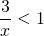### Solution

The best way to solve such an inequality is to make the right-hand side equal to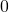. So we taketo the left-hand side and convert the fractions to the same denominator.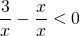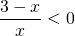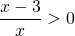For both numerator and denominator we draw a number line, as well as for the quotient: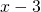--------------------(3)++++++--------------(0)++++++++++++++
quotient ++++(0)--------(3)++++++

The third line shows that the values ofthat satisfy the inequality are: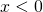or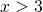0